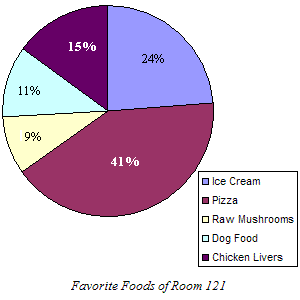# Circle Graphs

A circle graph is a way to organize data using the sectors of a circle.

Example:

Suppose you take a poll of the students in your class to find out their favorite foods, and get the following results:

• Pizza – $41%$
• Ice Cream – $24%$
• Raw Mushrooms – $9%$
• Dog Food – $11%$
• Chicken Livers – $15%$

Organize this data in a circle graph.

The data are given as percentages. To make the sectors the right size, you need to use the fact that there are $360$ degrees in a circle, and solve some proportions . For example, to find the number of degrees in the sector for chicken livers, you need to solve:

$\frac{15}{100}=\frac{x}{360}$

It turns out that $x=54$ , so you need to measure a $54$ degree sector to represent the portion of the class that loves chicken liver.